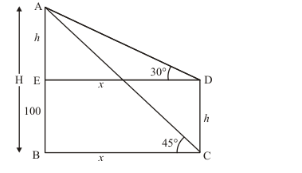# The angles of elevation of the top of a rock from the top and foot

Question:

The angles of elevation of the top of a rock from the top and foot of a 100 m high tower are respectively 30° and 45°. Find the height of the rock.

Solution:

Letbe the height of Rock which ism. and makes an angle of elevations 45° and 30° respectively from the bottom and top of tower whose height ism.

Let $A E=h \mathrm{~m}, B C=x \mathrm{~m}$ and $C D=100 \cdot \angle A C B=45^{\circ}, \angle A D E=30^{\circ}$

We have to find the height of the rock

We have the corresponding figure asSo we use trigonometric ratios.

In,

$\tan 45^{\circ}=\frac{A B}{B C}$

$\Rightarrow \quad 1=\frac{100+h}{x}$

$\Rightarrow \quad x=100+h$

Again in $\triangle A D E$

$\tan 30^{\circ}=\frac{A E}{D E}$

$\Rightarrow \quad \frac{1}{\sqrt{3}}=\frac{h}{x}$

$\Rightarrow 100+h=\sqrt{3} h$

$\Rightarrow \quad h=136.65$

$H=100+136.65$

$\Rightarrow \quad H=236.65$

Hence the height of rock is $236.65 \mathrm{~m}$.